# Selina Concise Mathematics Class 9 ICSE Solutions Rational and Irrational Numbers

## Selina Concise Mathematics Class 9 ICSE Solutions Rational and Irrational Numbers

APlusTopper.com provides step by step solutions for Selina Concise Mathematics Class 9 ICSE Solutions Chapter 1 Rational and Irrational Numbers. You can download the Selina Concise Mathematics ICSE Solutions for Class 9 with Free PDF download option. Selina Publishers Concise Mathematics for Class 9 ICSE Solutions all questions are solved and explained by expert mathematic teachers as per ICSE board guidelines.

Selina ICSE Solutions for Class 10 Maths Chapter 1 Rational and Irrational Numbers

Exercise 1(A)

Solution 1: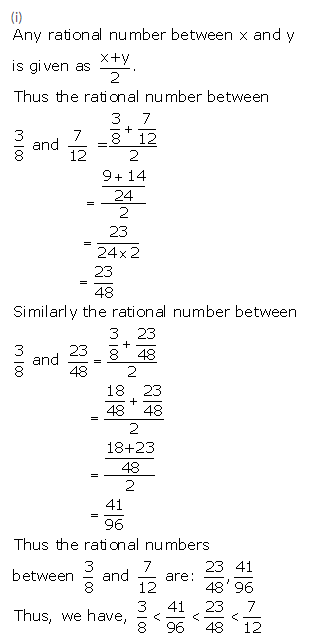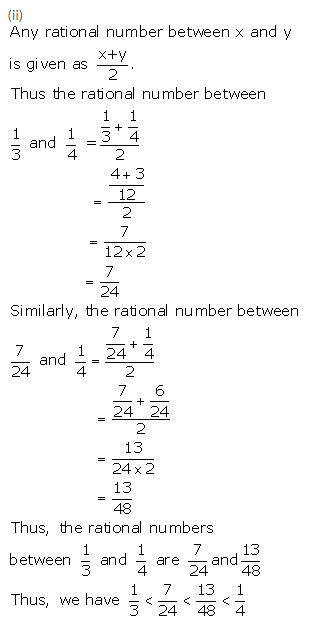Solution 2: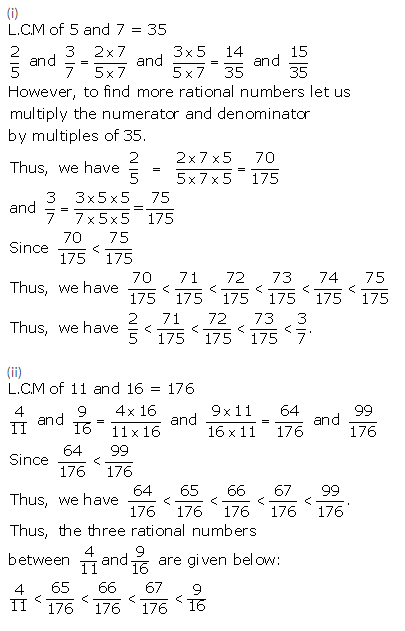Solution 3:Solution 4: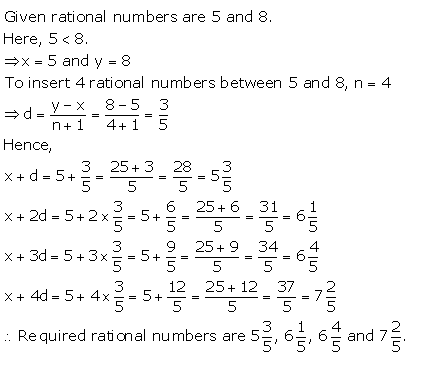Solution 5:Solution 6: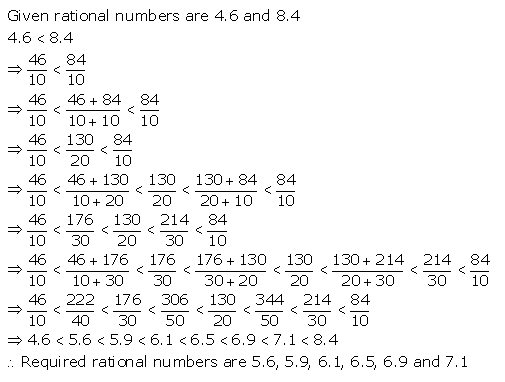Solution 7: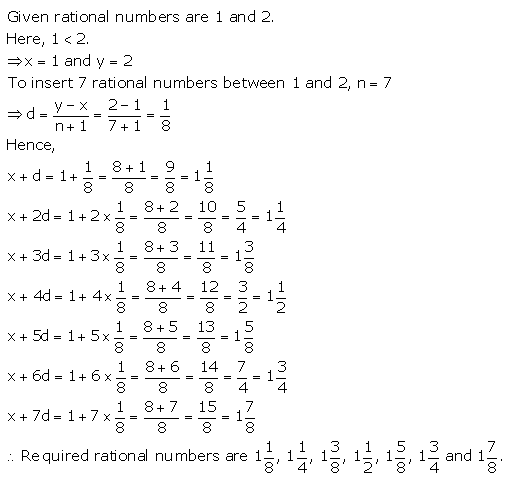Solution 8:Solution 9:Solution 10: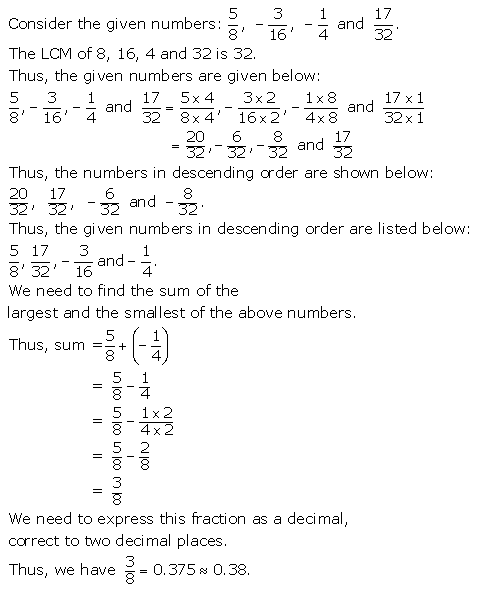Exercise 1(B)

Solution 1: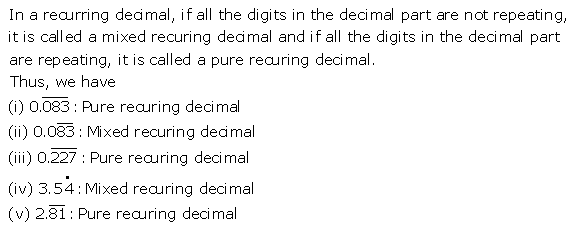Solution 2: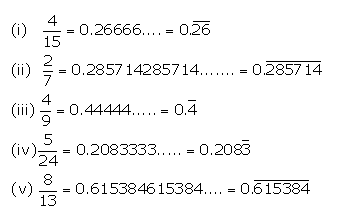Solution 3(i):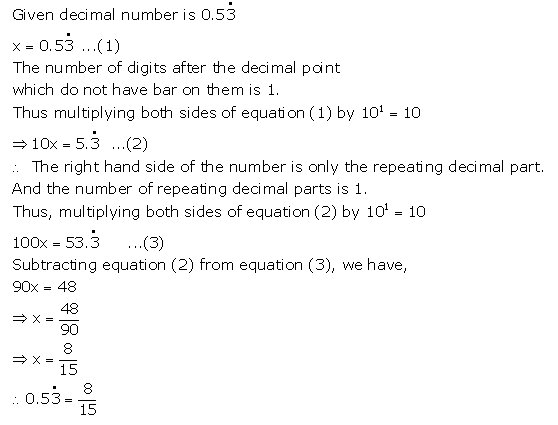Solution 3(ii):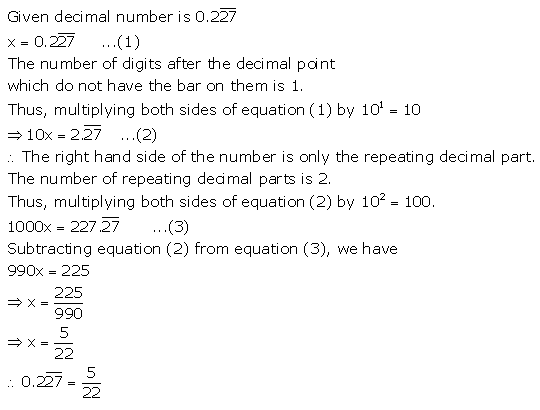Solution 3(iii):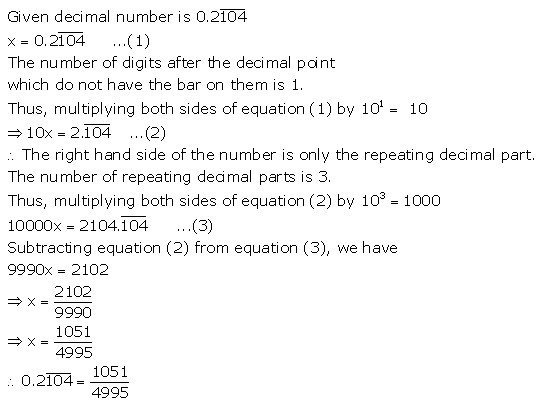Solution 3(iv):Solution 3(v):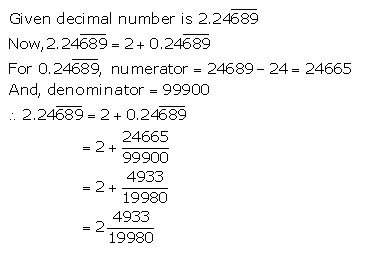Solution 3(vi):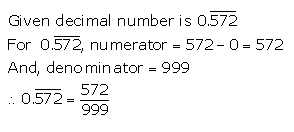Solution 3(vii):Solution 3(viii):Solution 4: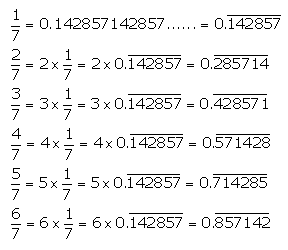Solution 5(i):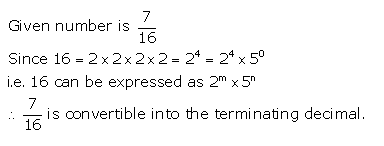Solution 5(ii):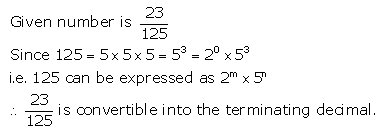Solution 5(iii):Solution 5(iv):Solution 5(v):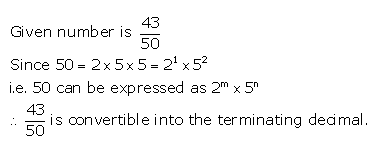Solution 5(vi):Solution 5(vii):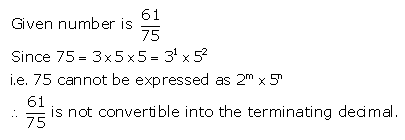Solution 5(viii):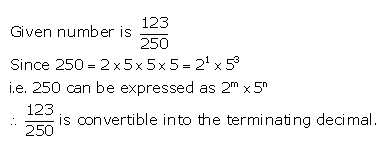Exercise 1(C)

Solution 1: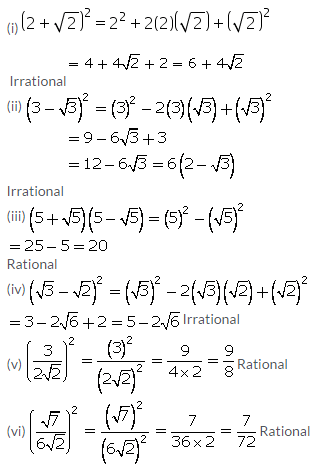Solution 2:Solution 3: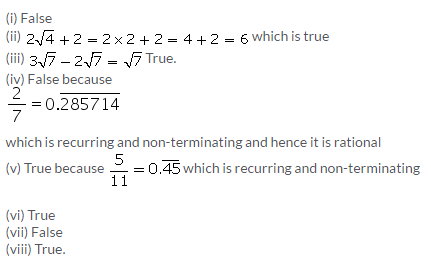Solution 4: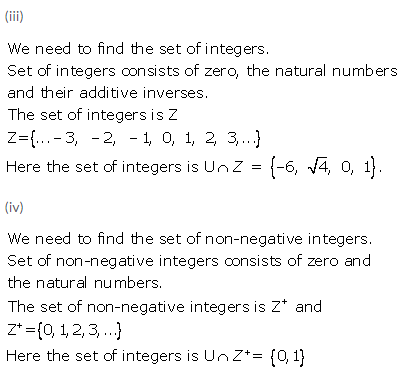Solution 5:Solution 6: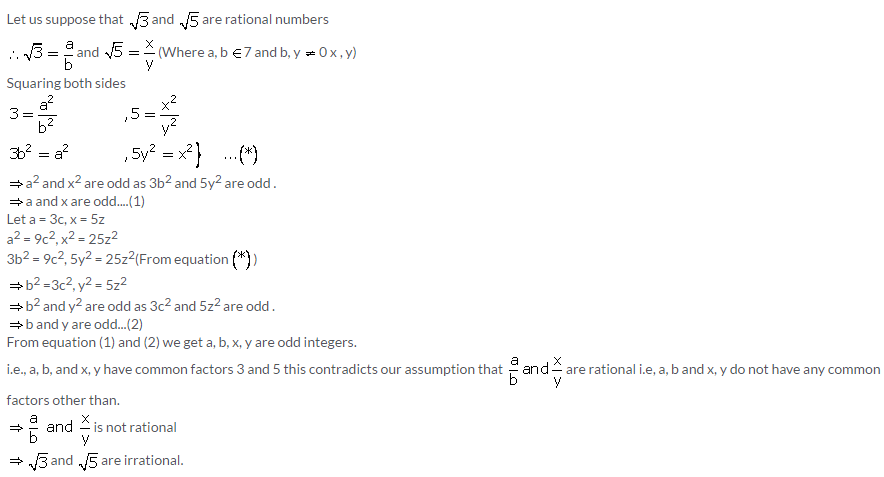Solution 7:Solution 8: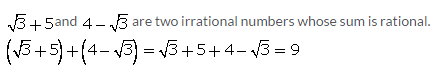Solution 9:Solution 10:Solution 11: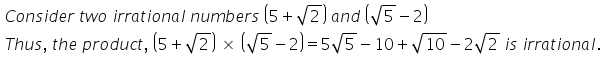Solution 12: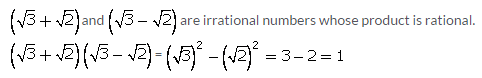Solution 13:Solution 14:Solution 15: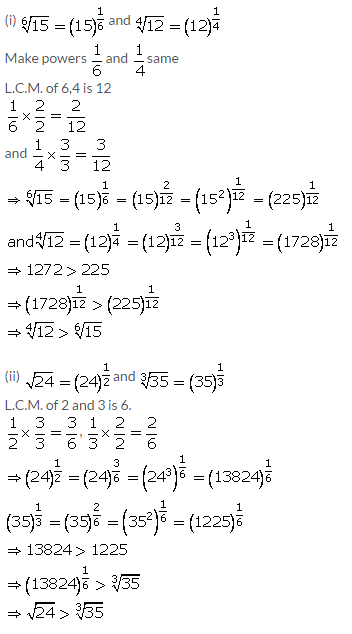Solution 16:Solution 17:Solution 18: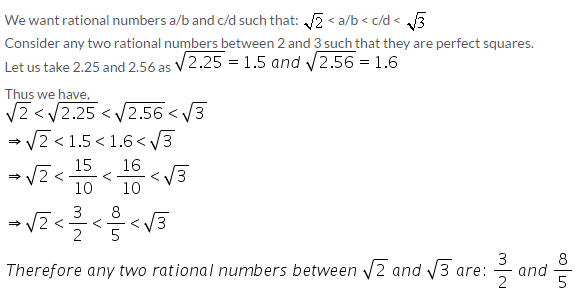Solution 19: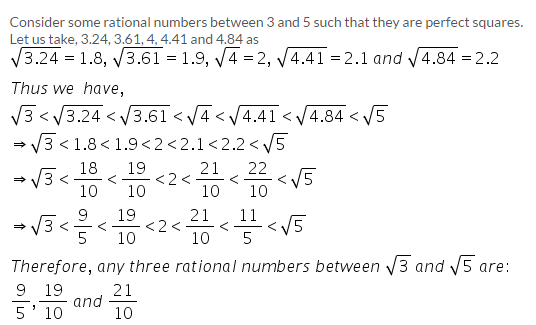Exercise 1(D)

Solution 1: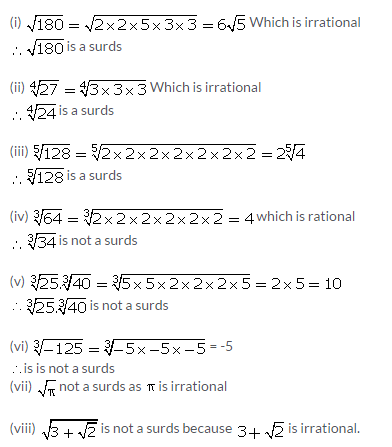Solution 2: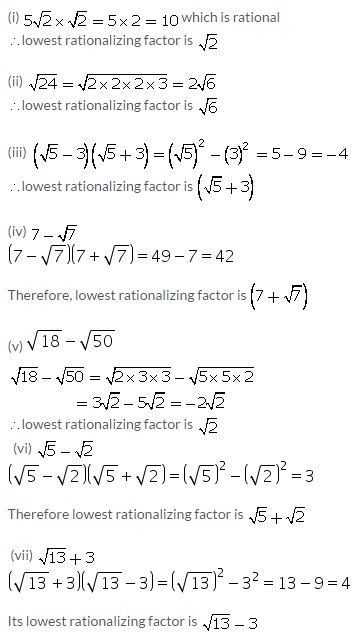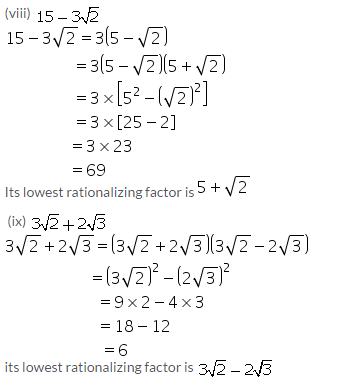Solution 3: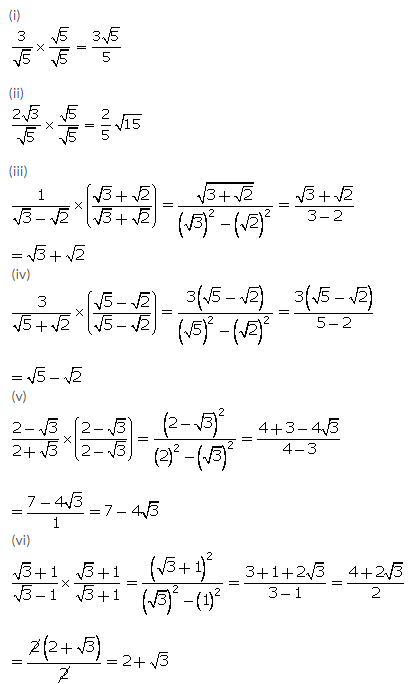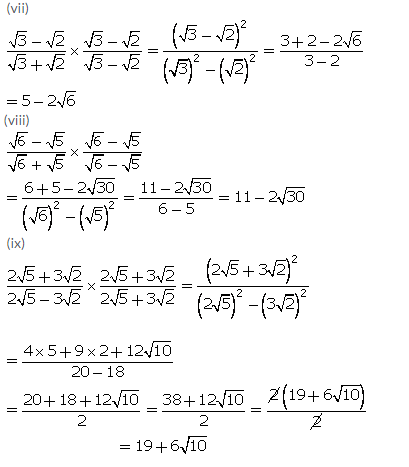Solution 4:Solution 5:Solution 6: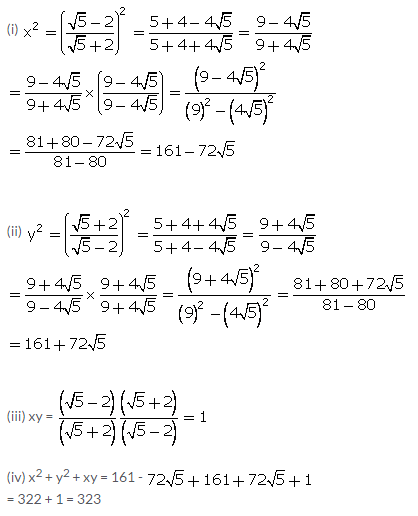Solution 7:Solution 8:Solution 9:Solution 10: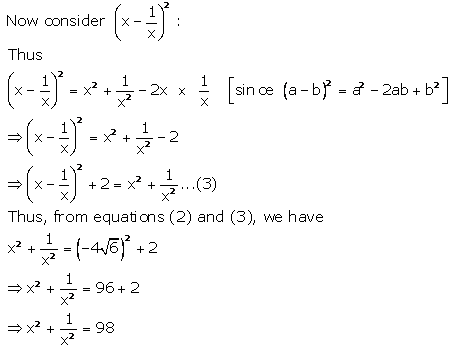Solution 11: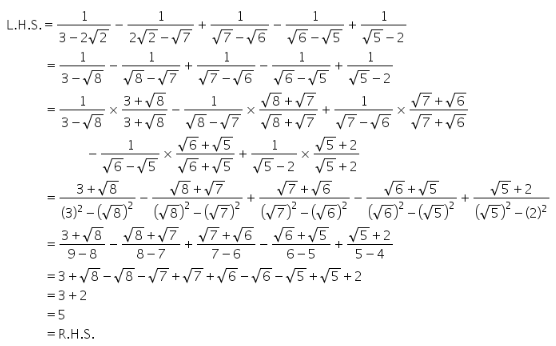Solution 12:Solution 13(i):Solution 13(ii):More Resources for Selina Concise Class 9 ICSE Solutions